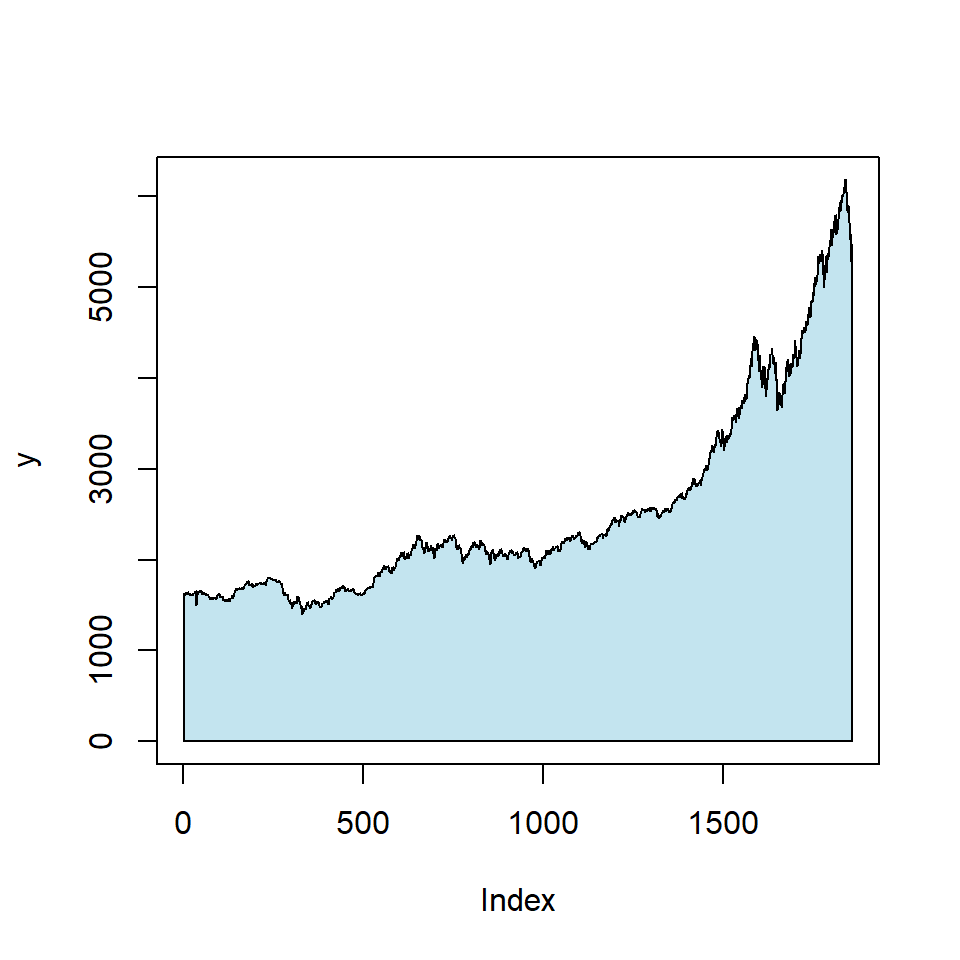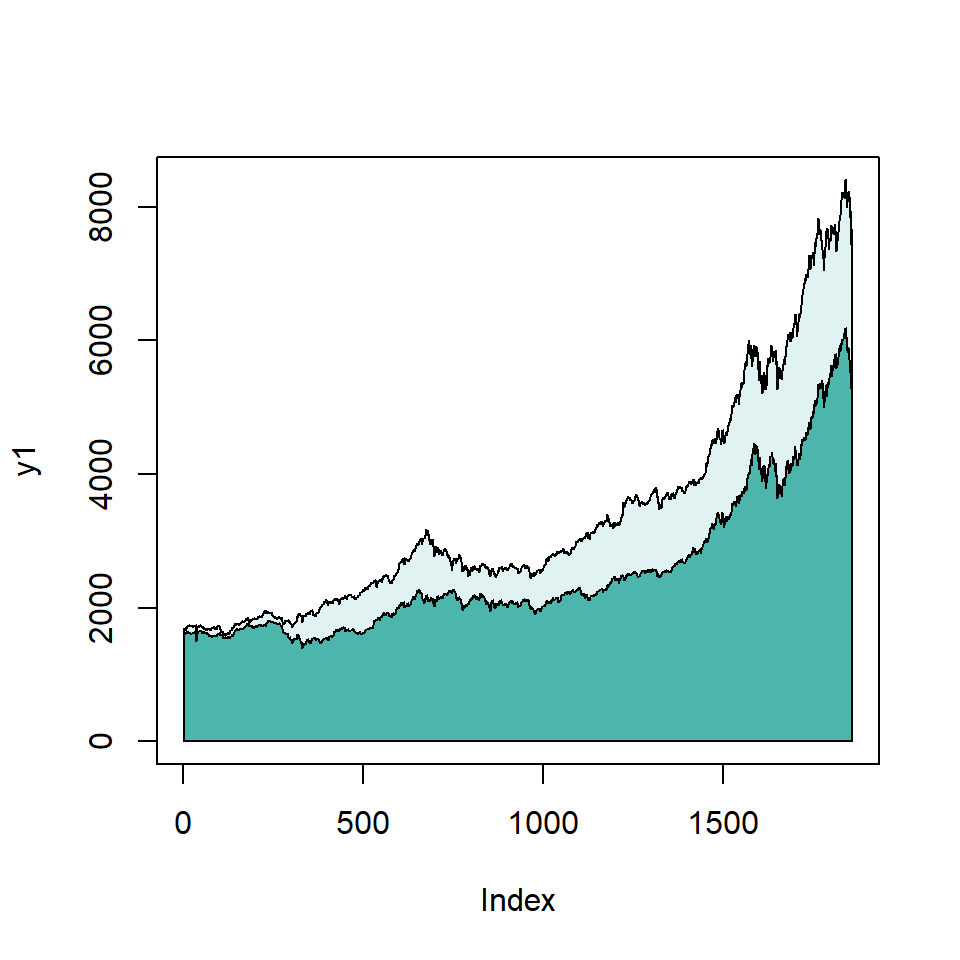# Area plot in R

## Sample data set

Consider the first two columns of the `EuStockMarkets` data frame which contains the closing prices for european stock indices.

``dat <- as.data.frame(EuStockMarkets[, 1:2])``

## Area chart of a single line

If you want to create an area chart for one of the variables you can make use of the `polygon` function or the `areaplot` function of the package of the same name. Note that using `areaplot` the Y-axis will start at 0.

``````dat <- as.data.frame(EuStockMarkets[, 1:2])

# First column
y <- dat[, 1]

# Area chart
plot(y, type = "l")

polygon(c(1, seq(y), length(y)), c(0, y, 0),
col = rgb(0.53, 0.79, 0.88, alpha = 0.5))

# Almost equivalent to:
# install.packages("areaplot")
library(areaplot)

areaplot(y, col = rgb(0.53, 0.79, 0.88, alpha = 0.5))``````## Area chart of several variables

If you want to create an area chart with several variables you can make use of the `polygon` function as shown below. Note that if one variable is always higher than the other you should plot it first. In addition, if the variables overlap you should use colors with transparency.

``````dat <- as.data.frame(EuStockMarkets[, 1:2])

# Second variable (because it is higher)
y1 <- dat\$SMI

# Plot
plot(y1, type = "l", ylim = c(0, max(dat)))

polygon(c(1, seq(y1), length(y1)), c(0, y1, 0),
col = "#E0F2F1")

# First variable
y2 <- dat\$DAX